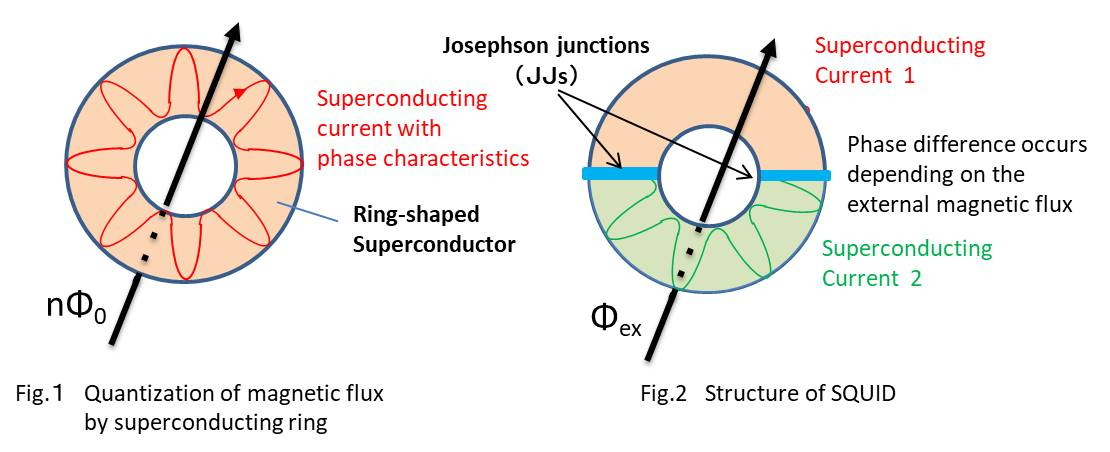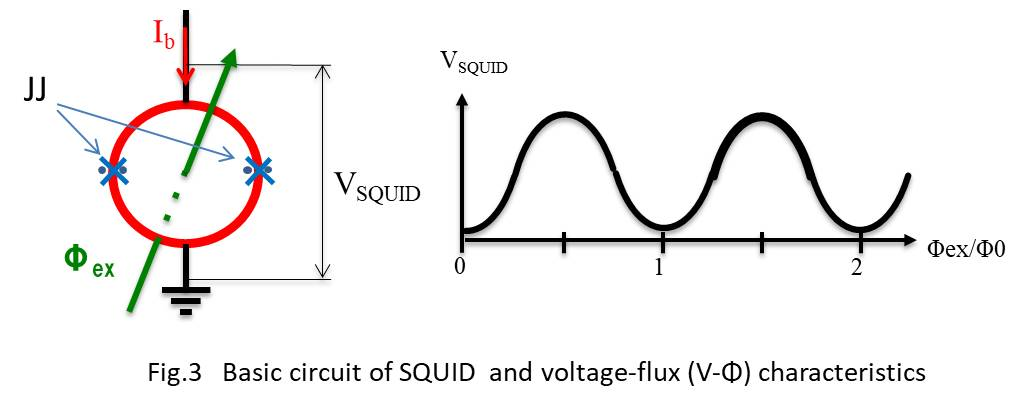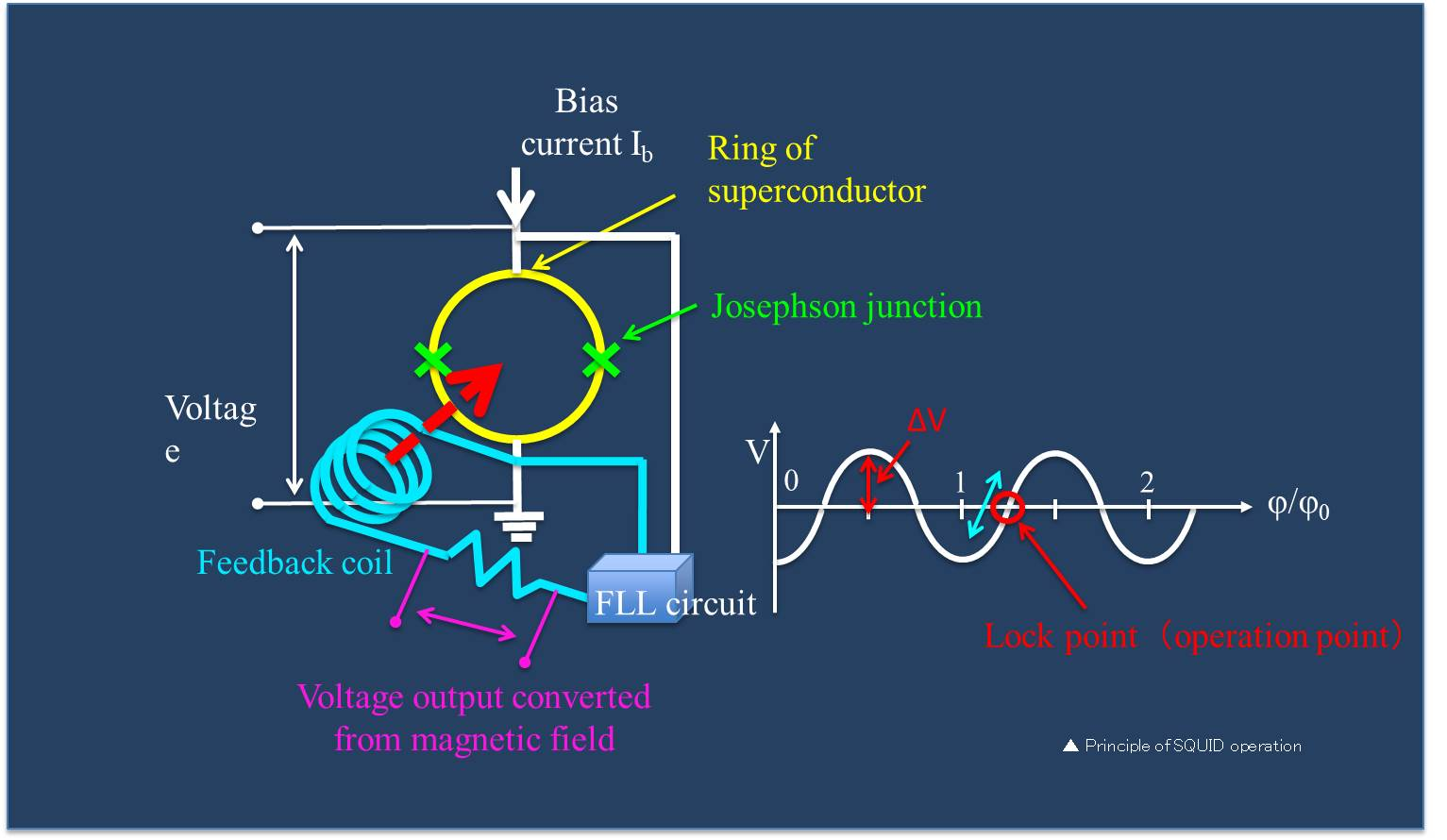# 超電導センサテクノロジー株式会社 SUSTEC（Superconducting Sensor Technology Corporation;　SUSTEC）

## Principle of superconducting quantum interference device (SQUID)

###### In the superconducting state, the electrical resistance becomes completely zero, and current flows even when the voltage is zero. This current is called superconducting current. Electrons (e-) are flowing in the normal current that has electrical resistance and requires voltage, but a pair of electrons (2e-) called a Cooper pair, are flowing in the superconducting current. All Cooper pairs are in the same energy state and have the property (phase) of a highly coherent wave. When the superconductor is made into a ring shape (closed loop), the circular superconducting current is subject to the condition that the phase matches when it makes one round. This is because if the phases do not match, the current will disappear and it will not exist. For this reason, only discontinuous values ​​are allowed. The magnetic flux corresponding to the circular current becomes a discontinuous value, which is called magnetic flux quantization (Fig. 1). Specifically, inside all superconductors, the magnetic flux is quantized to an integral multiple of the magnetic flux quantum Φ0 (2.07x10-15Wb). 　The SQUID magnetic sensor has a structure in which a ring-shaped superconductor is separated by two Josephson junctions (JJ) as shown in Fig.2. The Josephson junction has a structure in which two superconductors are weakly connected, and only a small amount of superconducting current (about several tens of μA) called Josephson current flows. Phase shifts are allowed at the Josephson junction, and the external magnetic flux (Φex) can take continuous values ​​within the SQUID ring. However, the phase difference of the superconducting current at the Josephson junction changes in response to the magnetic flux interlinking in the ring. If the phase difference is large, the Josephson current decreases, and the phase difference changes with the period of the magnetic flux quantum Φ0.###### When a constant bias current (Ib) slightly larger than the maximum Josephson current of SQUID is applied and the voltage (VSQUID) generated in SQUID is measured with respect to an external magnetic field, the voltage-magnetic flux characteristics shown on the right can be obtained. When the external magnetic flux is zero, the phase difference is the smallest and the generated voltage is low. As the external magnetic flux increases, the phase difference increases, and the Josephson current that flows at zero voltage decreases, so the voltage applied to SQUID increases. When the phase difference becomes 180 ° or more, the Josephson current ratio starts to increase as the next cycle approaches, and the voltage applied to SQUID decreases. As a result, the voltage output changes periodically (sine wave) with Φ0 as the period (Fig. 3). SQUID functions as a non-linear magnetic flux-voltage conversion device.## Linearization of SQUID signal by magnetic flux feedback control